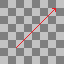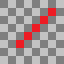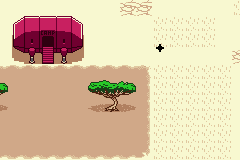# 8 directions movement like GBC/GBA Zelda

8 directions movement is complex to do in GBA as it involves traveling distances smaller than 1 pixel, we are sharing our solution, and we hope we can help you too.

What are the problems of movement in 8 directions?

The first problem is the speed on the diagonals, if you move a sprite by 1 pixel up and 1 to the left at the same time, for example, the space walked diagonally will be 1,414 times greater than when you are walking only in one of the 4 directions. This causes the speed when walking in diagonals to be faster than walking in the standard 4 directions.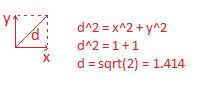To solve this, we need to ensure that the space walked diagonally is equal to 1, so by the Pythagorean Theorem, the x and y components should be approximately 0.707 (square root of 1/2).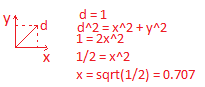The second problem is related to rounding. As the GBA doesn't know what a value smaller than a pixel is, there is always a rounding, and when the trajectory is "misaligned" as in the figure below, the rounding algorithm produces unwanted trajectories when walking in the diagonals.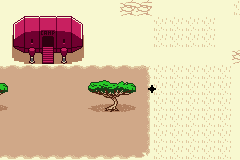To solve this, we need to round the current position of the sprite BEFORE doing the diagonal movement, this prevents the diagonal trajectory from being offset, and thus we have a reasonable diagonal movement, like in Zelda games from GBC/GBA